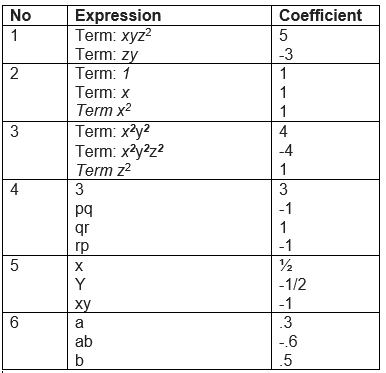# NCERT Solutions for Class 8 Maths Chapter 9 Algebraic Expressions and Identities Exercise 9.1

In this page we have NCERT Solutions for Class 8 Maths Chapter 9 Algebraic Expressions and Identities for Exercise 9.1. This exercise has question about Terms, Factors and Coefficient, Addition & subtraction of polynomials, type of polynomials and Like and Unlike Term. Hope you like them and do not forget to like , social share and comment at the end of the page.

## NCERT Solutions for Class 8 Maths Chapter 9 Exercise 9.1

Question 1
Identify the terms, their coefficients for each of the following expressions.
(i)5xyz2- 3zy
(ii)1 + x + x2
(iii)4x2y2- 4x2y2z2+ z2
(iv)3 - pq + qr - rp
(v) (x/2) -(y/2) -xy
(vi)0.3a - 0.6ab + 0.5bQuestion 2
Classify the following polynomials as monomials, binomials, trinomials. Which polynomials do not fit in any of these three categories?
x + y
1000
x + x2 + x3+ x4
7 + y + 5x
2y - 3y2
2y - 3y2+ 4y3
5x - 4y + 3xy
4z - 15z2
ab + bc + cd + da
pqr
p2q + pq2
2p + 2q
x + y: Binomial
1000: Monomial
x + x2+ x3+ x4:Polynomial
7 + y + 5x:Binomial
2y - 3y2: Binomial
2y - 3y2+ 4y3: Trinomial
5x - 4y + 3xy:Trinomial
4z - 15z2: Binomial
ab + bc + cd + da:Polynomial
pqr:Monomial
p2q + pq2: Binomial
2p + 2q:Binomial

Question 3
(i) ab - bc, bc - ca, ca - ab
(ii)a - b + ab, b - c + bc, c - a + ac
(iii)2p2q2- 3pq + 4, 5 + 7pq - 3p2q2
(iv)l2 + m2, m2+ n2, n2+ l2, 2lm + 2mn + 2nl
Answer (i) (ab - bc) + (bc - ca) + (ca-ab)
= ab + bc + ca - bc - ca - ab
= 0
(ii) (a - b + ab) + (b - c + bc) + (c - a + ac)
= a + b + c + ab + bc + ca - b - c - a
= ab + bc + ca
(iii) 2p2q2- 3pq + 4, 5 + 7pq - 3p2q2
= (2p2q2- 3pq + 4) + (5 + 7pq - 3p2q2)
= 2p2q2- 3p2q2 - 3pq + 7pq + 4 + 5
= - p2q2+ 4pq + 9
(iv) (l2+ m2) + (m2+ n2) + (n2+ l2) + (2lm + 2mn + 2nl)
=l2+ l2+ m2+ m2+ n2+ n2+ 2lm + 2mn + 2nl
=2l2+ 2m2+ 2n2+ 2lm + 2mn + 2nl

Question 4.
(a) Subtract 4a - 7ab + 3b + 12 from 2a - 9ab + 5b - 3
(b) Subtract 3xy + 5yz - 7zx from 5xy - 2yz - 2zx + 10xyz
(c) Subtract 4p2q - 3pq + 5pq2- 8p + 7q - 10
from 18 - 3p - 11q + 5pq - 2pq2+ 5p2q
Answer: While subtracting, we need to remember signs are reversed after -sign once bracket is opened
ie. + becomes - and - becomes +
Let solve the below question keeping that in mind
(a) (12a - 9ab + 5b - 3) - (4a - 7ab + 3b + 12)
= 12a - 9ab + 5b - 3 - 4a + 7ab - 3b - 12
=8a - 2ab + 2b - 15
(b) (5xy - 2yz - 2zx + 10xyz) - (3xy + 5yz - 7zx)
= 5xy - 2yz - 2zx + 10xyz - 3xy - 5yz + 7zx
= 2xy - 7yz + 5zx + 10xyz
(c) (18 - 3p - 11q + 5pq - 2pq2 + 5p2q) - (4p2q - 3pq + 5pq2 - 8p + 7q - 10)
= 18 - 3p - 11q + 5pq - 2pq2 + 5p2q - 4p2q + 3pq - 5pq2 + 8p - 7q + 10
= 28 + 5p - 18q + 8pq - 7pq2 - p2q

## Summary

1. NCERT Solutions for Class 8 Maths Chapter 9 Algebraic Expressions and Identities Exercise 9.1 has been prepared by Expert with utmost care. If you find any mistake.Please do provide feedback on mail. You can download the solutions as PDF in the below Link also
2. This chapter 9 has total 5 Exercise 9.1 ,9.2 ,9.3 ,9.4 and 9.5. This is the First exercise in the chapter.You can explore previous exercise of this chapter by clicking the link below